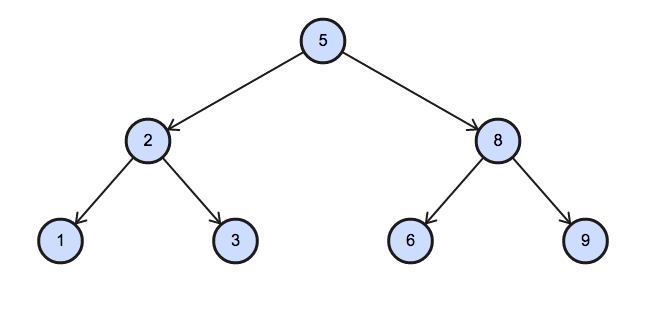## Algorithm

Problem Name: Sql - Binary Tree Nodes

In this HackerRank Functions in SQL problem solution,

You are given a table, BST, containing two columns: and P, where N represents the value of a node in Binary Tree, and P is the parent of N.Write a query to find the node type of Binary Tree ordered by the value of the node. Output one of the following for each node:

• Root: If node is root node.
• Leaf: If node is leaf node.
• Inner: If node is neither root nor leaf node.

Sample InputSample Output

``````1 Leaf
2 Inner
3 Leaf
5 Root
6 Leaf
8 Inner
9 Leaf
``````

Explanation

The Binary Tree below illustrates the sample:## Code Examples

### #1 Code Example with SQL

```Code - SQL```

``````
SELECT N, CASE WHEN P IS NULL THEN 'Root'
WHEN(SELECT COUNT(*) FROM BST WHERE P = A.N) > 0 THEN 'Inner'
ELSE 'Leaf'
END
FROM BST A
ORDER BY N;
``````
Copy The Code &